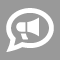#Blogs

## Division Blogs

Newest Most Active

In mathematics, word problems have been known to pose challenges for elementary school students, middle school students and even some high school students. In addition, a vast majority of students also have difficulties with solving problems with fractions. If we mix a word problem with a problem with fractions, then we end up getting an even tougher problem to solve. How can we expect those students who have not yet mastered language to make meaning of word problems? Let's dive right into a math word problem which will illustrate this.    Problem: Tashira has a piece of lace material that is 3/5 yard long. She used 2/3 of the material to make a quilt. How much did she use to make the quilt?   When a student reads this problem one of the questions she/he may ask is, "Where do I start?" The student may have difficulty with translating the word problem into its mathematical representation. The next difficulty is that if the student decides... read more

2: All even numbers are divisible by 2. Odd numbers are not divisible by 2. Any number that ends in a 0, 2, 4, 6, or 8 is even and can be divided by 2. 20, 34, 96, 48, and 102 are all divisible by 2. 31, 19, 27, 45, and 103 are not divisible by 2.   3: If all the digits in a number add up to 3, 6, or 9, the number is divisible by 3. For example, take the number 45. If you add the numbers 4 and 5 you get 9, which is divisible by 3. This means that the number 45 is divisible by 3. Another example is 57. If you add 5 and 7 you get 12. Now if you add the 1 and 2, you get 3. This means that the number 57 is divisible by 3. One last example is 19. If you add the 1 and 9, you get 10, which is not divisible by 3. This means that the number 19 is not divisible by 3. 15, 33, 63, 84, and 105 are all divisible by 3. 14, 32, 58, 71, and 103 are not divisible by 3.   4: Many (not all) even numbers are divisible by 4. Odd numbers... read more

Here are 48 of my favorite math words in 12 groups of 4. Each group has words in it that can be thought of at the same time or are a tool for doing math.   between on over in   each multiply of many   ratio divisions distribution compartments   limit neighborhood proximity boundary   infinite infitesmal mark differentiation   graph width height depth   circle sphere point interval   hyper extra spacetime dimensional   geometry proportion sketch spatial   four table cross squared   target rearrange outcome result   area volume space place   What are your favorite math words? If you aren't sure, search for "mathematical words" and pick a few.

Come with me on a journey of division. I have here a bag of M&Ms, which you and I and two of your friends want to share equally. I'm going to pour the bag out on the table and split it into four equal piles. For this example, “one bag” is our whole, and the best number to represent that whole would be the number of M&Ms in the bag. Let's say there were 32. If I split those 32 M&Ms into four equal piles and asked you how many were in one pile, you could certainly just count them. But a quicker way would be to take that 32 and divide it by the number of piles I'd made, which in this case is 4. You'd probably write that as: 32 ÷ 4 = 8 So there are 8 candies in each pile. Seems easy enough with a large number of M&Ms, right? But what if there were less candies – what if our “whole” was less than the entire bag? Well, for a while we'd be okay – if there were 16, for example, we'd do the same thing and come up with piles of 4 instead of... read more

## Stuck?

Find the perfect tutor and raise your grades.